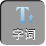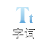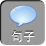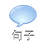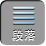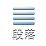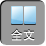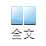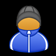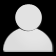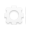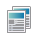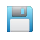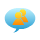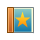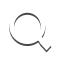-AA+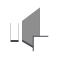1、下列代数式中，哪些是分式，哪些不是分式？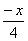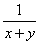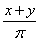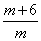2、已知分式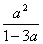.

1）当________时，分式的值等于 0.

2）当________时，分式无意义.

3）当________时，分式的值是正数.

4）当________时，分式的值是负数.

1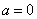2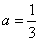3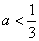4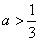.

1）当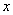______时，分式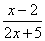的值为 0

2）当______时，分式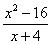的值为 0.

3）已知分式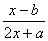，当= 2时，分式的值为零；当= -2时，分式没有意义.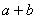的值

1= 2；（ 2= 4；（ 36

3、已知分式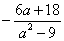的值是正整数，求整数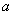.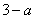= 1236.= 210-3.= -3时，无意义.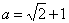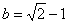时，代数式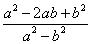的值是______________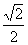4、已知分式 M=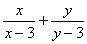.

1）若 x6且分式 M的值等于 4，求 y的值；

2）若 y4，当 x取哪些整数时， M的值是整数？

3）若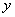均为正整数，写出使 M的值等于 2的所有的值

2）利用= 4时，代入进而利用整数的定义求出答案；

3）利用 M= 2，分别得出符合题意的答案.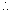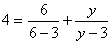2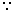= 4.M=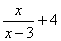= 0时， M= 4;= 2时， M= 2= 4时， M= 0= 6时， M= 6.

3均为正整数，写出使 M的值等于 2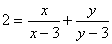所有的值为：= 2= 4= 4= 2.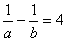，则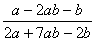的值是_________